Tetrahedron
 To the Main Page    "Mathematische Basteleien"

What is a Tetrahedron?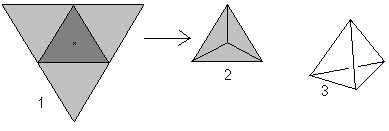A tetrahedron is a three-dimensional figure with four equilateral triangles. If you lift up three triangles (1), you get the tetrahedron in top view (2). Generally it is shown in perspective (3).

If you look at the word tetrahedron (tetrahedron means "with four planes"), you could call every pyramid with a triangle as the base a tetrahedron.

However the tetrahedron is the straight, regular triangle pyramid on this website.

Pieces of the Tetrahedron top
Height and area of a lateral triangle
Four equilateral triangles form a tetrahedron.... A triangle is picked out: The three heights intersect each other in one point as in every triangle. This is the centre of the triangle. The height can be calculated by the side a as h=sqr(3)/2*a using the Pythagorean theorem.
The heights also are medians and intersect with the ratio 2:1. That is used in the following calculations.

The area of the triangle is A=sqr(3)/4*a².

Space HeightThe height of the tetrahedron is between the centre of the basic triangle (1) and the vertex (2). For calculations you regard the so-called support triangle (3, yellow),   which is formed by one edge and two triangle heights. There is H=sqr(6)/3*a using the Pythagorean theorem.

Centre, Circumscribed Sphere, and Inscribed Sphere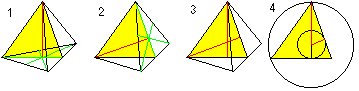The centre of a tetrahedron is the intersection of two space heights (1,2,3). It is centre of gravity, centre of the sphere through the four corners, and centre of the largest sphere, which still fits inside the tetrahedron (4).... You get two formulas for r and R with the help of the Pythagorean theorem (1) and H=R+r (2): There is r=sqr(6)/12*a , R=sqr(6)/4*a.

Angle
 ...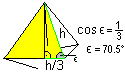... The angle of inclination (edge angle) between a lateral triangle and the base is seen in the yellow support triangle.  There is 70,5°.

Surface
 ....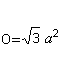..... The area of the base and the lateral faces form the surface O. There is O=4*A (triangle) = sqr(3)a².

VolumeIf you put a prism (1) with the volume A(triangle)*H around the tetrahedron and move the vertex to the corners of the prism three times (2,3,4), you get three crooked triangle pyramids with the same volume. They fill the prism (5).
Thus the volume of a triangle pyramid is (1/3)*A(triangle)*H.
There is V=sqr(2)/12*a³ for the tetrahedron.

Tetrahedal Numbers   top
 ...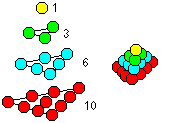... You can build a tetrahedron with layers of spheres. The number of the spheres in one layer is 1,3,6,10..., generally n(n+1)/2.  If you add the spheres layer by layer, you get the tetrahedral numbers 1,4,10,20,..., generally 1+3+6+10+...+n(n+1)/2=n(n+1)(n+2)/6.

 ...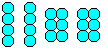... If you glue 20 marbles to two groups with four and two with six, you get a well known puzzle: You must form a pyramid with four pieces.
There are many similar puzzles.

Tetrahedron in the Cube top
Six face diagonals form a tetrahedron in the cube.
If you know the 3D-view, you can three-dimensionally look at the following two cube pairs.The volume of the tetrahedron is the third part of the volume of the cube.

If you draw a second tetrahedron and the lines of intersection, you get a penetration of two tetrahedrons.

The figure consists of the face diagonals and the connecting lines of the centres of the lateral squares of the original cube. The last ones form an octahedron.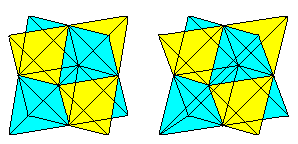Rings of Tetrahedrons  top
You can make paper tetrahedrons and stick an even number of them to a ring. The ring can continually twist inwards or outwards through the centre. This is a pretty toy. - These rings are also called kaleidocycles. You find more on my web page Kaleidocycles.

Tetrahedra on the Internet top

German

Christof Weber
Was hat dieser Körper mit Kugeln zu tun? (Reuleaux'sche Tetraeder)

Albert Kluge
Ein rotierender Tetraeder als Java-Applet

Georg Burkhard
Pyramide des Cestius, Rom (u.a.)

Gerd Müller
Platonische Körper in Stereodarstellung

H.B.Meyer (Polyeder aus Flechtstreifen)
Tetraeder

LANDRAT-LUCAS-GYMNASIUM Leverkusen
Sierpinski Tetraeder

ruhr-guide
Tetraeder Bottrop

Wikipedia
Tetraeder, Tetraeder (Bottrop), Tetrapode, Tetraederzahl

English

Eric W. Weisstein (World of Mathematics)
Tetrahedron

H.B.Meyer (Polyeder aus Flechtstreifen)
Tetrahedron

Joyce Frost and Peg Cagle (mathforum)
An Amazing, Space Filling, Non-regular Tetrahedron

Wikipedia
Tetrahedron, Tetrapod (structure)Tetrahedral number

Gail from Oregon, thank you for supporting me in my translation.

Feedback: Email address on my main page